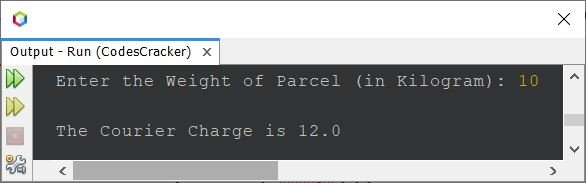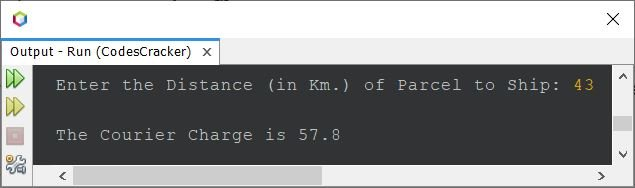# Java Program to Compute Courier Charges to Ship a Parcel

• The first, when we need to find the courier charge based on weight of the parcel to ship.
• The second, when we need to find the courier charge based on the distance of the address to ship a parcel
• The third and last, when we need to compute the courier charge based on distance and address, both

## Courier Charge based on Weight of the Parcel

The question is, write a Java program to compute courier charge based on parcel weight to ship. The charge based on weight must be calculated as per following criteria:

• When the weight of parcel is less than or equal to 5 Kilogram, then the courier charge will be \$6
• When the weight of the parcel is above 5 Kilogram, then there is an additional charge of \$1.2 for each extra Kilogram

The program given below is the answer to this question:

```import java.util.Scanner;

public class CodesCracker
{
public static void main(String[] args)
{
float weight, courierCharge;
Scanner scan = new Scanner(System.in);

System.out.print("Enter the Weight of Parcel (in Kilogram): ");
weight = scan.nextFloat();

if(weight<=5)
{
courierCharge = 6;
}
else
{
weight = weight - 5;
courierCharge = (float)(6 + (weight*1.2));
}
System.out.println("\nThe Courier Charge is " + courierCharge);
}
}```

Here is its sample run with user input 10 as the weight of parcel (in Kg.) to ship:Since the entered weight is 10, therefore for first 5 Kg., there is 6 charge, and then for remaining 5 Kg., there is an extra 1.2 charge per kg, that will be 1.2*5 or 6. Therefore total courier charge is 6+6, that is equal to 12.

## Courier Charge based on Distance of Address to Ship

This program is similar to previous one. The only difference is, this program calculates the courier charge based on the distance of parcel to ship. Here the distance means the distance of the address (customer's address) where the parcel is going to ship. The courier charge must be calculated as per following rates:

• When the distance is less than or equal to 6 Kilometer, then the courier charge will be \$4.2
• When the distance is above 6 Kilometer, then there is an additional charge of \$1.4 for each extra Kilometer

Here is the program based on above requirement:

```import java.util.Scanner;

public class CodesCracker
{
public static void main(String[] args)
{
float distance, courierCharge;
Scanner scan = new Scanner(System.in);

System.out.print("Enter the Distance (in Km.) of Parcel to Ship: ");
distance = scan.nextFloat();

if(distance<=6)
{
courierCharge = (float)4.2;
}
else
{
distance = distance - 6;
courierCharge = (float)(6 + (distance*1.4));
}
System.out.println("\nThe Courier Charge is " + courierCharge);
}
}```

The snapshot given below shows the sample run of above program with user input 43 as the distance of parcel:## Courier Charge based on both Weight and Distance

Now let's combine both the program. Because sometime we need to calculate the courier charge to ship a parcel based on the weight of parcel and the distance of parcel, both. Therefore here is the solution. In this program, I've used the same criteria as provided above for both.

```import java.util.Scanner;

public class CodesCracker
{
public static void main(String[] args)
{
float weight, distance, courierCharge;
Scanner scan = new Scanner(System.in);

System.out.print("Enter the Weight of Parcel (in Kg.): ");
weight = scan.nextFloat();
System.out.print("Enter the Distance of Parcel (in Km.): ");
distance = scan.nextFloat();

if(weight<=5)
courierCharge = 6;
else
{
weight = weight - 5;
courierCharge = (float)(6 + (weight*1.2));
}
if(distance<=6)
courierCharge = courierCharge + (float)4.2;
else
{
distance = distance - 6;
courierCharge = courierCharge + ((float)(4.2 + (distance*1.4)));
}
System.out.println("\nThe Courier Charge is " + courierCharge);
}
}```

Here is its sample run with user input 12 as weight and 32 as distance of the parcel to ship:Java Online Test

« Previous Program Next Program »